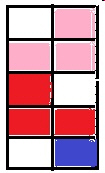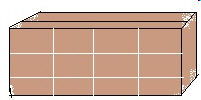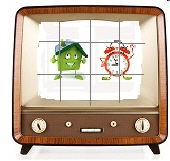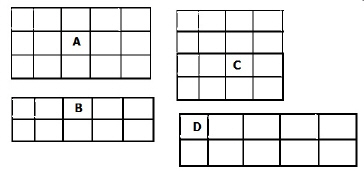3.MD.5.B.-Test1C A plane figure which can be covered by n unit squares
 Name:    3.MD.5.B.-Test1C A plane figure which can be covered by n unit squares

Multiple Response
Identify one or more choices that best complete the statement or answer the question.

1.

If an object is 13 square inches, what colors on this graph would show that?a. pink c. red b. blue

Completion
Complete each statement.

2.

If an object had an area of 14 square inches, how many square units would make up that area? PUT A NUMBER ONLY.

3.

How many square units does the object have? PUT A NUMBER ONLY.4.

How many square units does the side of this box have?  PUT A NUMBER ONLY.5.

How many square units does the TV screen have?  PUT A NUMBER ONLY.6.

If an object had 2 sq. units X 9 sq. units, how many square units would that object have?

7.

Which graph below shows 16 square units? USE AN UPPER CASE LETTER.